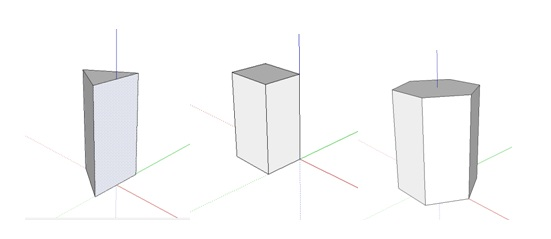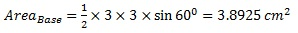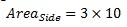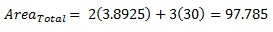# How to Find the Surface Area of a Prism

## What is a Prism

A prism is a polyhedron, in which is a solid object consisting of two congruent (similar in shape and equal in size) polygonal faces with their identical edges connected by rectangles. The polygonal face is known as the base of the prism, and the two bases are parallel to each other. However, it is not necessary that they are exactly positioned above each other. If they are positioned exactly above each other, then the rectangular sides and the base meets at right angles, then, the prism is known as a right-angled prism.Any of these shapes can be referred to as a prism.

## How to find the surface area of a prism: Method

A prism contains at least 5 surfaces. Moreover, if the prism is irregular, most likely the area of each surface has to be calculated separately and has to be added to get the total surface area. Nevertheless, in a regular prism with familiar geometry this problem is a little simpler.

Prism has two base surfaces and n number of rectangles connecting these surfaces. In some cases, the shape is irregular, and the area varies from one surface to another. Then, we can find the area of the prism by following formula.

Total Area=2[Area of the Base]+[Area of all Sides, the Rectangles]

If the bases are a regular polygon, the sides or the rectangles become similar and same in size. Therefore, it is enough to calculate the area of a single base and the area of a single rectangle. Assuming a regular prism geometry and for a n sided polygon as the base, the total area becomes.

Total Area=2[Area of the Base]+n[Area of a Side, the Rectangle]

Triangular prisms are the commonly used type of prisms, and considering an equilateral triangular prism with we can modify the above formula into,

Total Area of a Triangular Prism=2[1/2 ah]+3[al]

Where the length of a side of the prism is l, h is the perpendicular height of the triangle with side a.

## How to find the surface area of a prism: Example

1. A prism has a cross-sectional area of an equilateral triangle with 3cm sides. If the prism is 10cm long find the total area of the prism.
• Find the area of the base

Base is an equilateral triangle with 3cm. Therefore, the area of the triangle is,• Find the area of a side.

A side is rectangular in shape and 10cm in length and 3cm in width, therefore, the area of a single side,• There are 3 sides and two bases in a triangular Prism, therefore, the total area of the prism is,